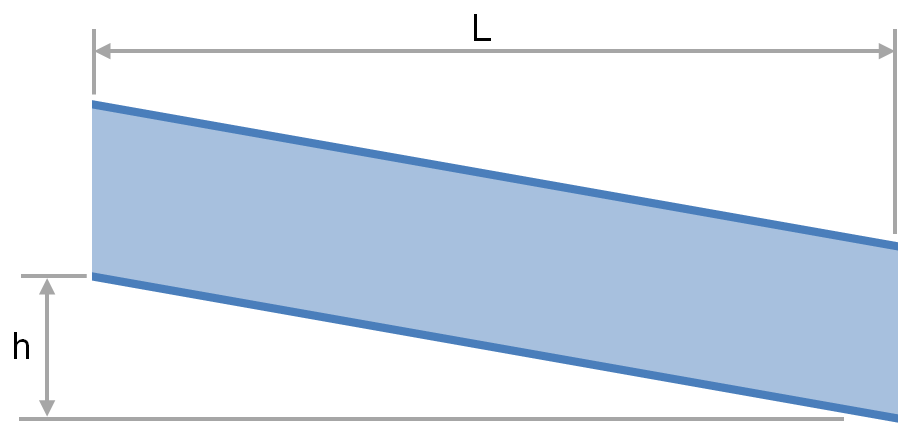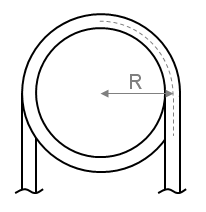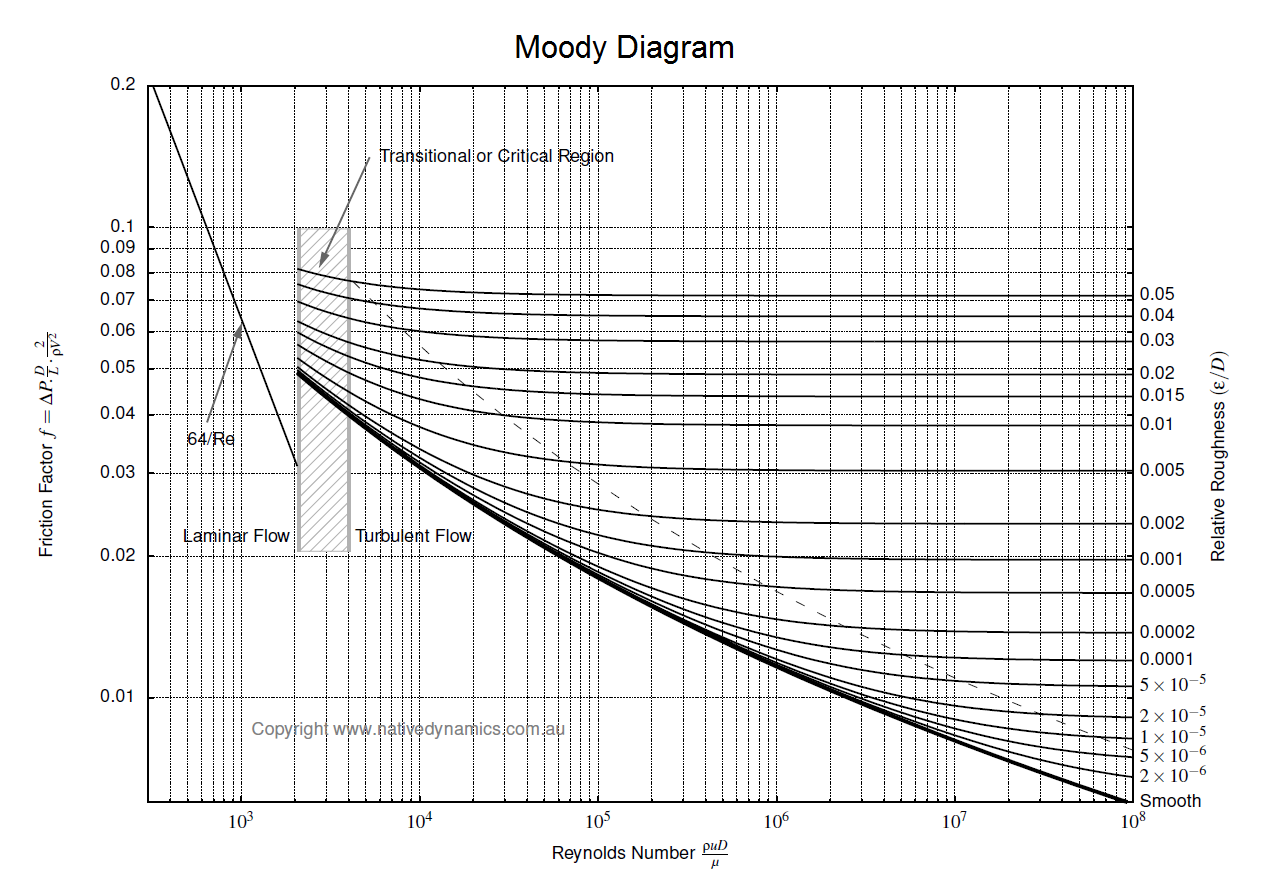f
Neutrium

# Roughness

Flow in Open Channels and Partially Filled PipesThe transport of fluid under gravity is often achieved using partially filled pipes, channels, flumes, ditches and streams. To determine the slope and elevation change required or the flow rate that is achievable one must be able to calculate the head loss and friction factor. This article provides relationships for the calculation of head loss and friction factor for fluids flowing via these conduits.

Friction Factor for Flow in Coils and Curved PipeIn order to determine the pressure drop in a pipe or coil the friction factor must first be calculated. This article presents the equations which may be used to determine the friction factor in coils and curved pipe.

Pressure Loss in PipeTo determine the pressure loss or flow rate through pipe knowledge of the friction between the fluid and the pipe is required. This article describes how to incorporate friction into pressure loss or fluid flow calculations. It also outlines several methods for determining the Darcy friction factor for rough and smooth pipes in both the turbulent and laminar flow regime. Finally this article discusses which correlation for pressure loss in pipe is the most appropriate.

Thermal Contact Resistance

Thermal contact conductance is required to evaluate heat transfer through the interface of two materials and is dependent on a range of material and interface properties. This article provides a brief summary of these properties and a list of thermal contact conductance for a selection of metal-metal pairs.